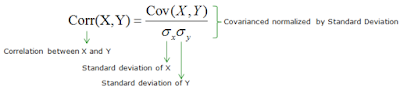Online Machine Learning Quiz

100+ Objective Machine Learning Questions. Lets see how many can you answer?

Start Quiz

## Friday, 8 February 2019

### Difference between Covariance and Correlation in Machine Learning

Covariance and Correlation are two important concepts of Mathematics (especially of Probability and Statistics) which are heavily used in Machine Learning mainly for Data Analysis and Data Wrangling.

Dimensionality Reduction in Machine Learning mainly depends upon Covariance and Correlation among different variables or features in the dataset. For example, PCA (Principal Component Analysis) algorithm uses Correlation concept for Feature Extraction.

Covariance and Correlation describe the relationship and inter-dependence between two variables. Both Covariance and Correlation depict how the change in one variable affects the change in another variable? Relationship between two variables or features can be positive relationship, negative relationship or there could be no relationship at all.

Difference between Covariance and Correlation

1. Correlation between the two variables is a normalized version of the Covariance

To calculate the Correlation between random variables X and Y, we need to divide the Covariance of X and Y by the product of the Standard Deviation of X and the Standard Deviation of Y.